PHP Training

### Data Types and Operators

#### PHP Conditional Statements

Conditional statements are used to perform different actions based on different conditions.

Very often when you write code, you want to perform different actions for different conditions. You can use conditional statements in your code to do this.

In PHP we have the following conditional statements:

• if statement - executes some code if one condition is true
• if...else statement - executes some code if a condition is true and another code if that condition is false
• if...elseif....else statement - executes different codes for more than two conditions
• switch statement - selects one of many blocks of code to be executed

#### PHP - The if Statement

The if statement executes some code if one condition is true.

### Syntax

if (condition) {
code to be executed if condition is true
;
}

The example below will output "Have a good day!" if the current time (HOUR) is less than 20:

#### PHP - The if...else Statement

The if....else statement executes some code if a condition is true and another code if that condition is false.

### Syntax

if (condition) {
code to be executed if condition is true;
} else {
code to be executed if condition is false;
}

The example below will output "Have a good day!" if the current time is less than 20, and "Have a good night!" otherwise:

### Example

<?php
\$t = date("H");

if (\$t < "20") {
echo "Have a good day!";
} else {
echo "Have a good night!";
}
?>
//Output:
Have a good day!

#### PHP - The if...elseif....else Statement

The if....elseif...else statement executes different codes for more than two conditions.

### Syntax

if (condition) {
code to be executed if this condition is true;
} elseif (condition) {
code to be executed if this condition is true;
} else {
code to be executed if all conditions are false;
}

The example below will output "Have a good morning!" if the current time is less than 10, and "Have a good day!" if the current time is less than 20. Otherwise it will output "Have a good night!":

### Example

<?php
\$t = date("H");

if (\$t < "10") {
echo "Have a good morning!";
} elseif (\$t < "20") {
echo "Have a good day!";
} else {
echo "Have a good night!";
}
?>
//Output:

The hour (of the server) is 15, and will give the following message:

Have a good day!

#### The PHP switch Statement

Use the switch statement to select one of many blocks of code to be executed .

### Syntax

switch (n) {
case label1:
code to be executed if n=label1;
break;
case label2:
code to be executed if n=label2;
break;
case label3:
code to be executed if n=label3;
break;
...
default:
code to be executed if n is different from all labels;
}

This is how it works: First we have a single expression n (most often a variable), that is evaluated once. The value of the expression is then compared with the values for each case in the structure. If there is a match, the block of code associated with that case is executed. Use break to prevent the code from running into the next case automatically. The default statement is used if no match is found.

### Example

<?php
\$favcolor = "red";

switch (\$favcolor) {
case "red":
echo "Your favorite color is red!";
break;
case "blue":
echo "Your favorite color is blue!";
break;
case "green":
echo "Your favorite color is green!";
break;
default:
echo "Your favorite color is neither red, blue, nor green!";
}
?>
//Output:

#### PHP Loops

Often when you write code, you want the same block of code to run over and over again in a row. Instead of adding several almost equal code-lines in a script, we can use loops to perform a task like this.

In PHP, we have the following looping statements:

• while - loops through a block of code as long as the specified condition is true
• do...while - loops through a block of code once, and then repeats the loop as long as the specified condition is true
• for - loops through a block of code a specified number of times
• foreach - loops through a block of code for each element in an array

#### The PHP while Loop

The while loop executes a block of code as long as the specified condition is true.

### Syntax

while (condition is true) {
code to be executed;
}

The example below first sets a variable \$x to 1 (\$x = 1). Then, the while loop will continue to run as long as \$x is less than, or equal to 5 (\$x <= 5). \$x will increase by 1 each time the loop runs (\$x++):

### Example

<?php
\$x = 1;

while(\$x <= 5) {
echo "The number is: \$x <br>";
\$x++;
}
?>
//Output:
The number is: 1
The number is: 2
The number is: 3
The number is: 4
The number is: 5

#### The PHP do...while Loop

The do...while loop will always execute the block of code once, it will then check the condition, and repeat the loop while the specified condition is true.

### Syntax

do {
code to be executed;
} while (condition is true);

The example below first sets a variable \$x to 1 (\$x = 1). Then, the do while loop will write some output, and then increment the variable \$x with 1. Then the condition is checked (is \$x less than, or equal to 5?), and the loop will continue to run as long as \$x is less than, or equal to 5:

### Example

<?php
\$x = 1;

do {
echo "The number is: \$x <br>";
\$x++;
} while (\$x <= 5);
?>
//Output:
The number is: 1
The number is: 2
The number is: 3
The number is: 4
The number is: 5

Notice that in a do while loop the condition is tested AFTER executing the statements within the loop. This means that the do while loop would execute its statements at least once, even if the condition is false the first time.

The example below sets the \$x variable to 6, then it runs the loop, and then the condition is checked :

### Example

<?php
\$x = 6;

do {
echo "The number is: \$x <br>";
\$x++;
} while (\$x <= 5);
?>
//Output:
The number is: 6

#### The PHP for Loop

The for loop is used when you know in advance how many times the script should run.

PHP for loops execute a block of code a specified number of times.

### Syntax

for (init counter; test counter; increment counter) {
code to be executed;
}

Parameters:

• init counter: Initialize the loop counter value
• test counter: Evaluated for each loop iteration. If it evaluates to TRUE, the loop continues. If it evaluates to FALSE, the loop ends.
• increment counter: Increases the loop counter value

The example below displays the numbers from 0 to 10:

### Example

<?php
for (\$x = 0; \$x <= 10; \$x++) {
echo "The number is: \$x <br>";
}
?>
//Output:
The number is: 0
The number is: 1
The number is: 2
The number is: 3
The number is: 4
The number is: 5
The number is: 6
The number is: 7
The number is: 8
The number is: 9
The number is: 10

#### The PHP foreach Loop

The foreach loop works only on arrays, and is used to loop through each key/value pair in an array.

### Syntax

foreach (\$array as \$value) {
code to be executed;
}

For every loop iteration, the value of the current array element is assigned to \$value and the array pointer is moved by one, until it reaches the last array element.

The following example demonstrates a loop that will output the values of the given array (\$colors):

### Example

<?php
\$colors = array("red", "green", "blue", "yellow");

foreach (\$colors as \$value) {
echo "\$value <br>";
}
?>
//Output:
red
green
blue
yellow

#### PHP Functions

The real power of PHP comes from its functions; it has more than 1000 built-in functions.

Besides the built-in PHP functions, we can create our own functions.

A function is a block of statements that can be used repeatedly in a program.

A function will not execute immediately when a page loads.

A function will be executed by a call to the function.

#### Create a User Defined Function in PHP

A user defined function declaration starts with the word "function":

### Syntax

function functionName() {
code to be executed;
}

Note: A function name can start with a letter or underscore (not a number).

Tip: Give the function a name that reflects what the function does!

Function names are NOT case-sensitive.

In the example below, we create a function named "writeMsg()". The opening curly brace ( { ) indicates the beginning of the function code and the closing curly brace ( } ) indicates the end of the function. The function outputs "Hello world!". To call the function, just write its name:

### Example

<?php
function writeMsg() {
echo "Hello world!";
}

writeMsg(); // call the function
?>
//Output:
Hello world!

#### PHP Function Arguments

Information can be passed to functions through arguments. An argument is just like a variable.

Arguments are specified after the function name, inside the parentheses. You can add as many arguments as you want, just separate them with a comma.

The following example has a function with one argument (\$fname). When the familyName() function is called, we also pass along a name (e.g. Jani), and the name is used inside the function, which outputs several different first names, but an equal last name:

### Example

<?php
function familyName(\$fname) {
echo "\$fname Refsnes.<br>";
}

familyName("Jani");
familyName("Hege");
familyName("Stale");
familyName("Kai Jim");
familyName("Borge");
?>
//Output:
Jani Refsnes.
Hege Refsnes.
Stale Refsnes.
Kai Jim Refsnes.
Borge Refsnes.

The following example has a function with two arguments (\$fname and \$year):

### Example

<?php
function familyName(\$fname, \$year) {
echo "\$fname Refsnes. Born in \$year <br>";
}

familyName("Hege", "1975");
familyName("Stale", "1978");
familyName("Kai Jim", "1983");
?>
//Output:
Hege Refsnes. Born in 1975
Stale Refsnes. Born in 1978
Kai Jim Refsnes. Born in 1983

#### PHP Default Argument Value

The following example shows how to use a default parameter. If we call the function setHeight() without arguments it takes the default value as argument:

### Example

<?php
function setHeight(\$minheight = 50) {
echo "The height is : \$minheight <br>";
}

setHeight(350);
setHeight(); // will use the default value of 50
setHeight(135);
setHeight(80);
?>
//Output: The height is : 350
The height is : 50
The height is : 135
The height is : 80

#### PHP Functions - Returning values

To let a function return a value, use the return statement:

### Example

<?php
function sum(\$x, \$y) {
\$z = \$x + \$y;
return \$z;
}

echo "5 + 10 = " . sum(5, 10) . "<br>";
echo "7 + 13 = " . sum(7, 13) . "<br>";
echo "2 + 4 = " . sum(2, 4);
?>
//Output:
5 + 10 = 15
7 + 13 = 20
2 + 4 = 6

#### Dynamic Function Calls

It is possible to assign function names as strings to variables and then treat these variables exactly as you would the function name itself. Following example depicts this behaviour.

```<html>

<title>Dynamic Function Calls</title>

<body>

<?php
function sayHello() {
echo "Hello<br />";
}

\$function_holder = "sayHello";
\$function_holder();
?>

</body>
</html>```

This will display following result −

```Hello
```

### PHP Workflow

( 0 Review )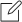### Course Code :

02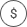### Price :

5000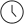### Duration :

30 days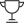### Lectures :

10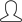### Students :

10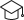### Certificate of Completion### Manish Kumar Shrivastava

Web Developer

My name is Ruth. I grew up and studied in…